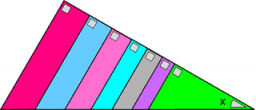# Find all

Find all right-angled triangles whose side lengths form an arithmetic sequence.

Result

n = (Correct answer is: ∞)### Step-by-step explanation:Did you find an error or inaccuracy? Feel free to write us. Thank you!

Showing 1 comment:
Dr Math
there are infinity solutions. for leg lenght a,  difference AP is a/3. So right triangle has sides: a, a+a/3, a+2/3a

So all triangles are similar to triangle 3-4-5 and has length of sides: a ; 4/3 a ; 5/3*a

Tips for related online calculators
Are you looking for help with calculating roots of a quadratic equation?
Need help calculating sum, simplifying, or multiplying fractions? Try our fraction calculator.
Do you have a linear equation or system of equations and looking for its solution? Or do you have a quadratic equation?